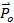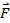### Network Solution Methodology

AFT Fathom makes use of standard matrix solution techniques (Jeppson 1976Jeppson, R. W., Analysis of Flow in Pipe Networks, Ann Arbor Science Publishers, Ann Arbor, MI, 1976.). The method is known as the H-Equation method, where H, the piezometric head, is solved for at each junction by forcing continuity of flow through each connecting pipe. Simultaneously, the head loss across each pipe is updated based on the flow balance information. The flow rate and head are solved in an inner-outer loop algorithm, where the flow is guessed, the head loss is calculated consistent with that guess, and the flow is updated according to the new pressure drop information. The Newton-Raphson method is employed to refine each successive solution, resulting in a sparse square matrix that is solved during each solution pass.

The concepts of pressure and hydraulic gradeline (HGL, also called piezometric head) are related but use different frameworks for considering pipe system behavior. The HGL includes both the static and elevational effects of pressure. The relationship between the two is given by Equation 1:(1)

where z is the elevation and P is the static pressure. Each concept offers certain advantages.

The solution technique makes use of the continuity and one-dimensional momentum equations. AFT Fathom uses pressure and mass flow rate as the unknown parameters because they are more fundamental in nature than the head and volumetric flow rate used by Jeppson and thus extend more naturally to mechanical and chemical systems.

In the following discussion, subscripts denote values at junctions. Thus, Pi represents the static pressure at junction i. Double subscripts denote values along pipes connecting two junctions. Thus, ij represents the mass flow rate in the pipe connecting junctions i and j.

Application of the law of mass conservation to each junction yields:(2)

where n is the number of pipes connected to junction i. Equation 2 states that the net mass flow rate into each junction must sum to zero.

The basic equation for pipe pressure drop due to friction can be expressed with the Darcy-Weisbach equation:(3)

where ΔPf is the frictional pressure loss. The total pressure change between junctions is given by the momentum equation in the form of the Bernoulli equation:(4)

The static (normal), P, and stagnation (pressure if all kinetic energy were converted isentropically into pressure), Po, pressures are related as follows:(5)

Substituting Equation 5 into 4Solving for the frictional pressure drop for a constant area pipes yields:(6)

where i and j denote upstream and downstream junction values.

The definition of mass flow rate is:(7)

Combining Equations 3 and 6 and substituting for velocity, V, using Equation 7 gives the mass flow for each pipe:(8)

where Rij' is the effective flow resistance in the pipe and the subscript ij refers to the pipe connecting junctions i and j.(9)

Note that R' is not the same as R, which is the resistance used in the Output Control window and is expressed by the following equation:Substituting Equation 8 into Equation 2 results in the equation to be applied to each junction i:(10)

where n is the number of pipes connected to junction i.

To be completely general, Equation 10 should be written for junction i:(11)

to allow for application of boundary condition flow rates to a boundary junction node.

Equation 11 as applied to each junction in the network represents the system of equations that need to be solved to determine the stagnation pressure at each junction. To solve this system, the Newton-Raphson method is employed. In the Newton-Raphson method, new values for each unknown are calculated based on the previous value and a correction that uses the first derivative of the function.

In this instance the function that Newton-Raphson is applied to would be of the form:(12)

The method involves finding all the junction stagnation pressures, Po,i , that cause all of the Fi to go to zero, thus satisfying Equation 11 at all junctions.

When applied to a system of equations, the Jacobian matrix contains all the required derivative information to employ the Newton-Raphson technique. The Jacobian, JF, is given by:The column matrixcontains the stagnation pressure at each junction, and column matrixcontains the F values (Equation 12) at each junction. The updated solutions forare obtained from the following Newton-Raphson equation:(13)

Traditional implementations of the Newton-Raphson method require initial guesses at the solution before the Solver can begin. This often results in the situation where you have to know the solution ahead of time in order to solve for it. To avoid placing this burden on the user, AFT Fathom uses a proprietary method for generating first guesses. This method is usually sufficient to get the solution going in the right direction, especially for systems that don't have highly non-linear features.

However, there are times when AFT Fathom's first guess does not lead to a converged solution. In these cases, AFT Fathom provides you with the option of specifying your own initial guesses of flow and pressure.